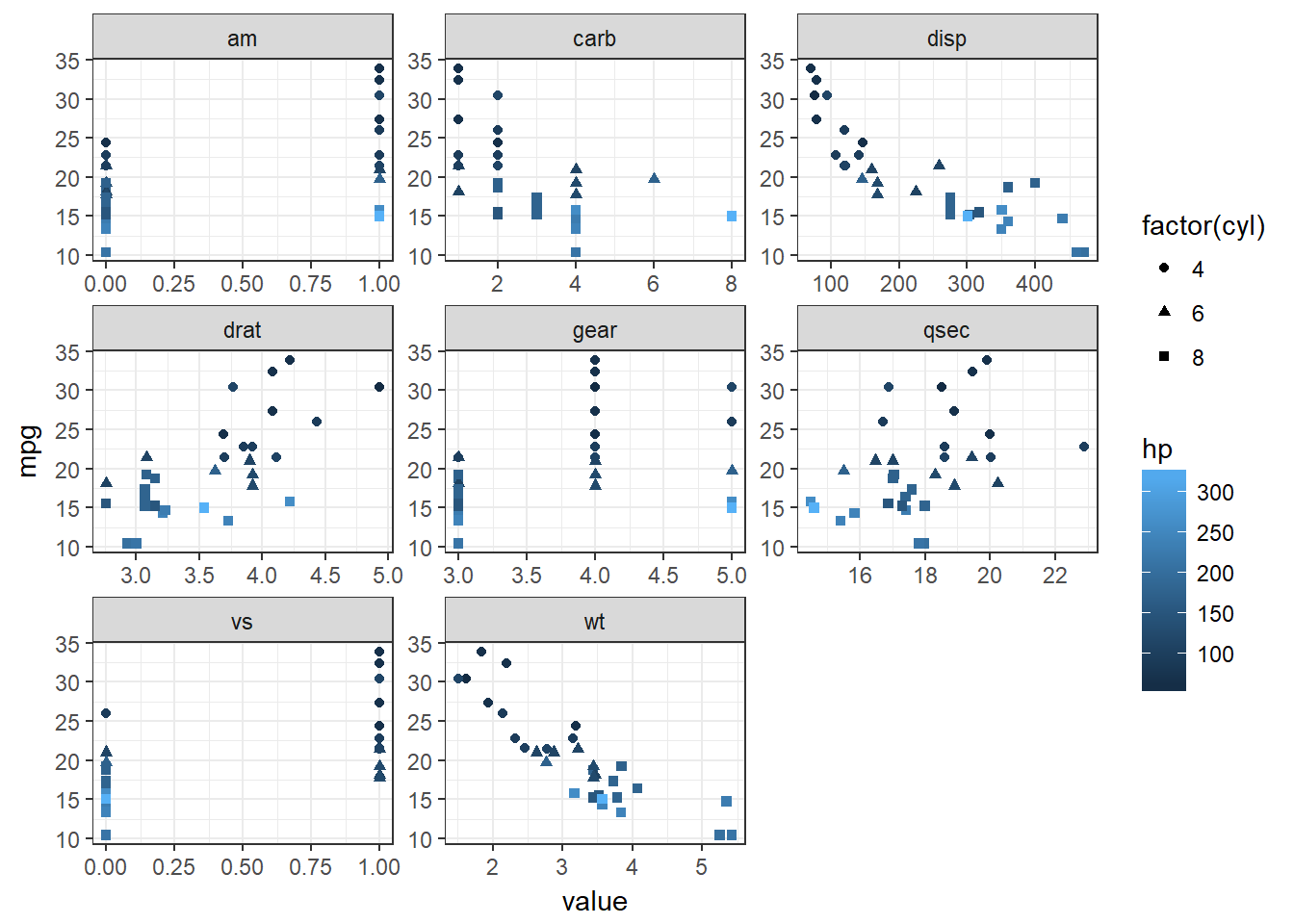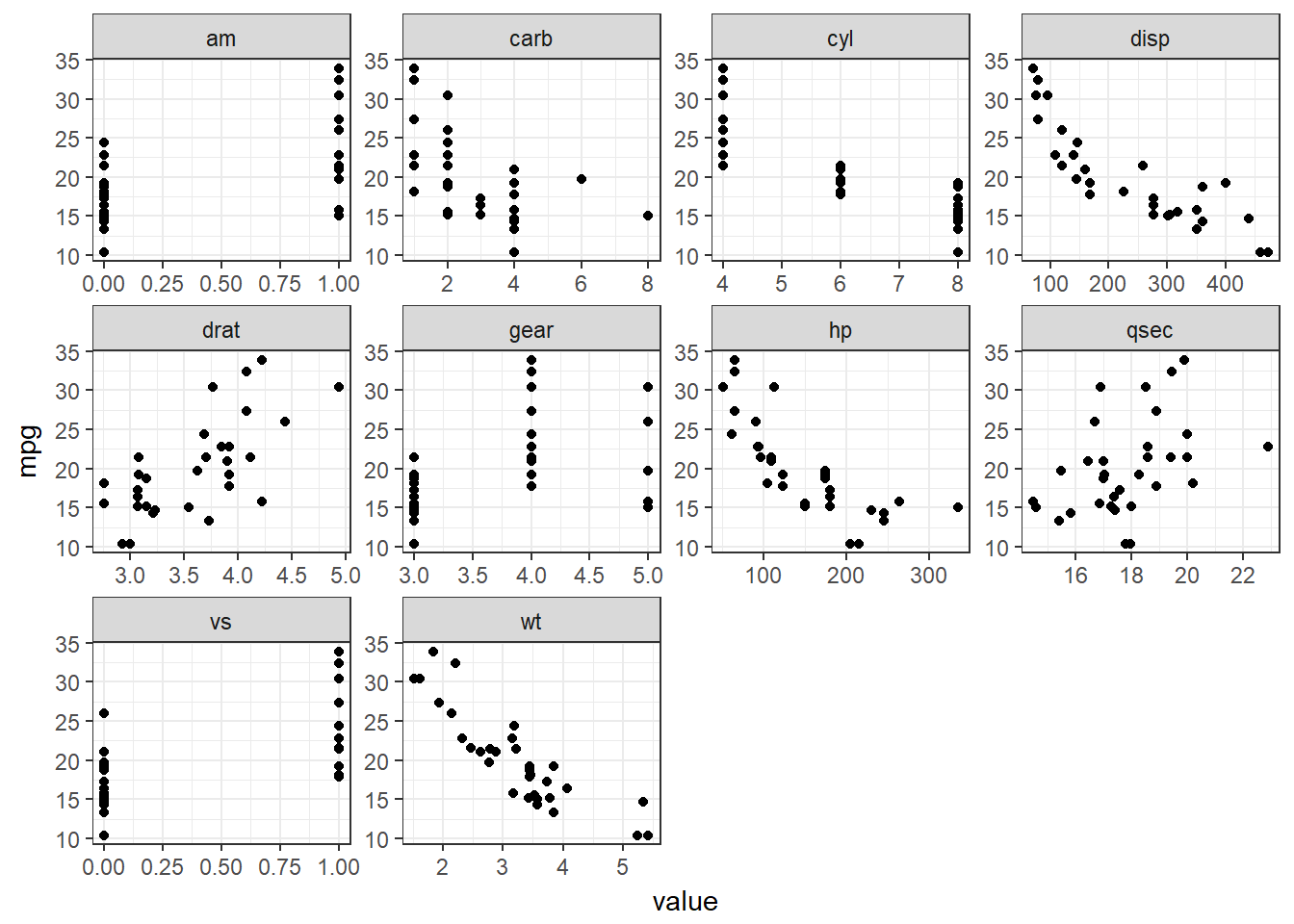## Introduction - the Finished Plot

See how variables relate to many others? Here is an example. This shows a separate scatter plot panel for each of many variables against mpg; all points are coloured by hp and the shapes refer to cyl.

``````mtcars %>% gather(-mpg, -hp, -cyl, key = "var", value = "value") %>%
ggplot(aes(x = value, y = mpg, color = hp, shape = factor(cyl))) + geom_point() +
facet_wrap(~ var, scales = "free") + theme_bw()``````Now we will go through the code to understnad how this is created.

## Tidying the data

Make use of the `facet_wrap()` in `ggplot2`, but doing so requires some careful data prep. Assuming our data frame has all the variables we are interested in, the first step is to get our data into a tidy form that is suitable for plotting.

Do this using `gather()` from the `tidyr` package. We get all of our variables using mtcars as our example data set:

``mtcars %>% gather() %>% head()``
``````##   key value
## 1 mpg  21.0
## 2 mpg  21.0
## 3 mpg  22.8
## 4 mpg  21.4
## 5 mpg  18.7
## 6 mpg  18.1``````

This gives us a key column with the variable names and a value column with their corresponding values. This works well if we only want to plot each variable by itself (to get univariate information).

We are interested in visualizing multivariate information with a focus on one or two variables. Start with the bivariate case. Within `gather()`, we drop our variable of interest (mpg):

``mtcars %>% gather(-mpg, key = "var", value = "value") %>% head()``
``````##    mpg var value
## 1 21.0 cyl     6
## 2 21.0 cyl     6
## 3 22.8 cyl     4
## 4 21.4 cyl     6
## 5 18.7 cyl     8
## 6 18.1 cyl     6``````

Now we have an mpg column with the values of mpg repeated for each variable in the var column. The value column contains the values corresponding to the variable in the var column. This simple extension is how we can use `gather()` to get our data into shape.

### Creating the plot

We want a scatter plot of mpg with each variable in the var column whose values are in the value column. Creating a scatter plot is handled by `ggplot()` and `geom_point()`. Getting a separate panel for each variable is handled by `facet_wrap()`. We also want the scales for each panel to be “free”. Otherwise, ggplot will constrain them all the be equal, which does not make sense for plotting different variables. Also add theme_bw().

``````mtcars %>% gather(-mpg, key = "var", value = "value") %>%
ggplot(aes(x = value, y = mpg)) +  geom_point() + facet_wrap(~ var, scales = "free") + theme_bw()``````### Extracting more than one variable

We can layer other variables into these plots. Say we want to colour the points based on hp. To do this, we also drop hp within `gather()` and then include it in the plotting stage:

``mtcars %>% gather(-mpg, -hp, key = "var", value = "value") %>% head()``
``````##    mpg  hp var value
## 1 21.0 110 cyl     6
## 2 21.0 110 cyl     6
## 3 22.8  93 cyl     4
## 4 21.4 110 cyl     6
## 5 18.7 175 cyl     8
## 6 18.1 105 cyl     6``````
``````mtcars %>% gather(-mpg, -hp, key = "var", value = "value") %>%
ggplot(aes(x = value, y = mpg, color = hp)) + geom_point() + facet_wrap(~ var, scales = "free") + theme_bw()``````